# Common Excel Functions in SQL

My first job out of college was in insurance analytics. I learned a lot about insurance and a lot about Excel. Excel, and now Google Sheets, is a pivotal tool in analytical and operational jobs, but it’s not as popular for data engineers. The way you think in databases and Excel are different, and tool switching can be just as bad for productivity as context switching, so I do everything in SQL/Snowflake when I can. I export to Google Sheets as a last result (usually when the stakeholder requests it).

For that same reason, I often have stakeholders who, when they have to do something in Snowflake, “speak” Excel to me; they want to know how to do a vlookup, get subtotals, or do an if statement. This guide attempts to bridge the communication gap between analysts and data engineers by translating Excel to SQL. Now perhaps we will have more to discuss than just the fact that people think we’re all data scientists. Not everyone who works with data is a data scientist. I’m talking to you, Linkedin recruiters and non-technical friends.

## IF Statement (and other IFs)

### IF

An IF statement is powerful; it helps you do everything from fix typos to group data. In the example below, I create a “Mac?” column that is True when the cat is Mac.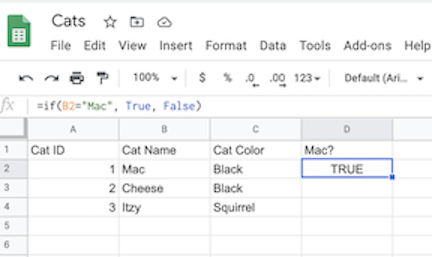The formula for this is:
`=if(C2="Mac", True, False)`
If we imagine we had the same cat table in Snowflake, the equivalent formula would be:

``````SELECT
*,
IFF(cat_name = 'Mac', True, False) AS is_mac
FROM cat_table;
``````

In a database, double quotes (") also denotes a database object, such as a column name. A literal string (Mac) is put in single quotes. Other than that, the function is quite similar.

Snowflake reference for IFF

### IFS

The complexity comes in once we there are multiple IF statements to evaluate. To determine whether the cat is one of the twins, I have to allow that the cat could be either Mac or Cheese (they actually had another litter mate who we could not adopt). I also want to label them Twin1 (Mac) and Twin2 (Cheese). In Excel, the function is:

`=if(C2="Mac", "Twin1", if(C2="Cheese", "Twin2", "Not Twin"))`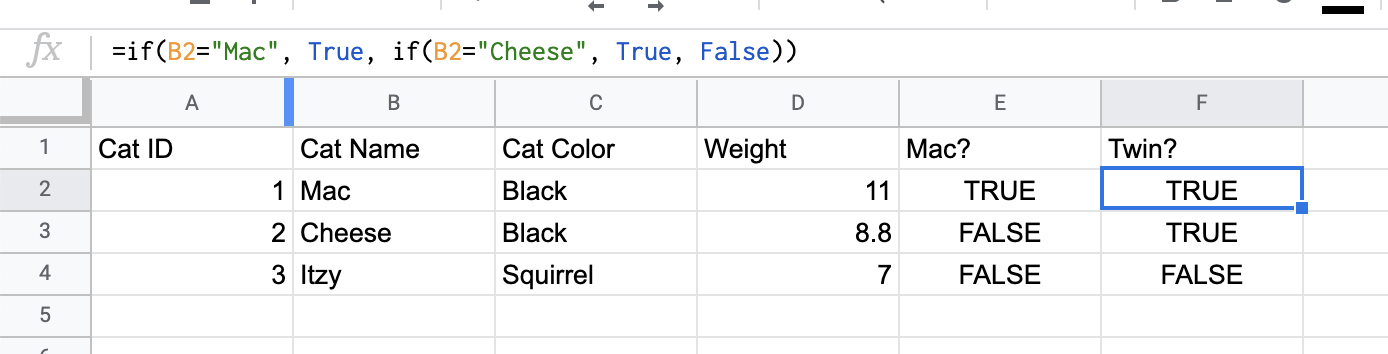Notice how in order to have more than two outcomes (True/False versus Twin1/Twin2/Not Twin), we need to embed another IF statement into the false_value. This gets unreadable really quickly. Luckily, in SQL you can do what’s called a `case` statement or a `case when` statement. A `case` statement for this would be:

``````SELECT
*,
CASE
WHEN cat_name = 'Mac'
THEN 'Twin1'
WHEN cat_name = 'Cheese'
THEN 'Twin2'
ELSE 'Not Twin'
END AS twin_number
FROM cat_table;
``````

Maybe it is the spacing or the lack of parenthesis, but I find this more readable and intuitive. Notice the single quotes again. The ELSE clause is optional; without explicitly giving a value to those that do not fall in a category, they will be NULL. I often write “ELSE NULL END” to be explicit, but this is technically redundant. Similar to Excel, the resulting value of the CASE WHEN is whichever evaluates as true first. In our example, a cat cannot be named Mac and Cheese, so let’s say we had this statement:

``````SELECT
*,
CASE
WHEN cat_name = 'Mac'
THEN 'Twin1'
WHEN cat_color = 'Black'
THEN 'Twin2'
ELSE 'Not Twin'
END AS twin_number
FROM cat_table;
``````

Although Mac is black, he will have twin_number of Twin1 since `cat_name = 'Mac'` evaluates to true first.

Note: I tried to use the Excel function IFS but I couldn’t figure out how to include a default value (“Not Twin”):
`=ifs(C2="Mac", "Twin1", F2="Black", "Twin2")`

### SUMIF

Now that we know who the twins are, we can sum only the twins' weights. In Excel, we would do: `=sumif(I2:I4,True,G2:G4)`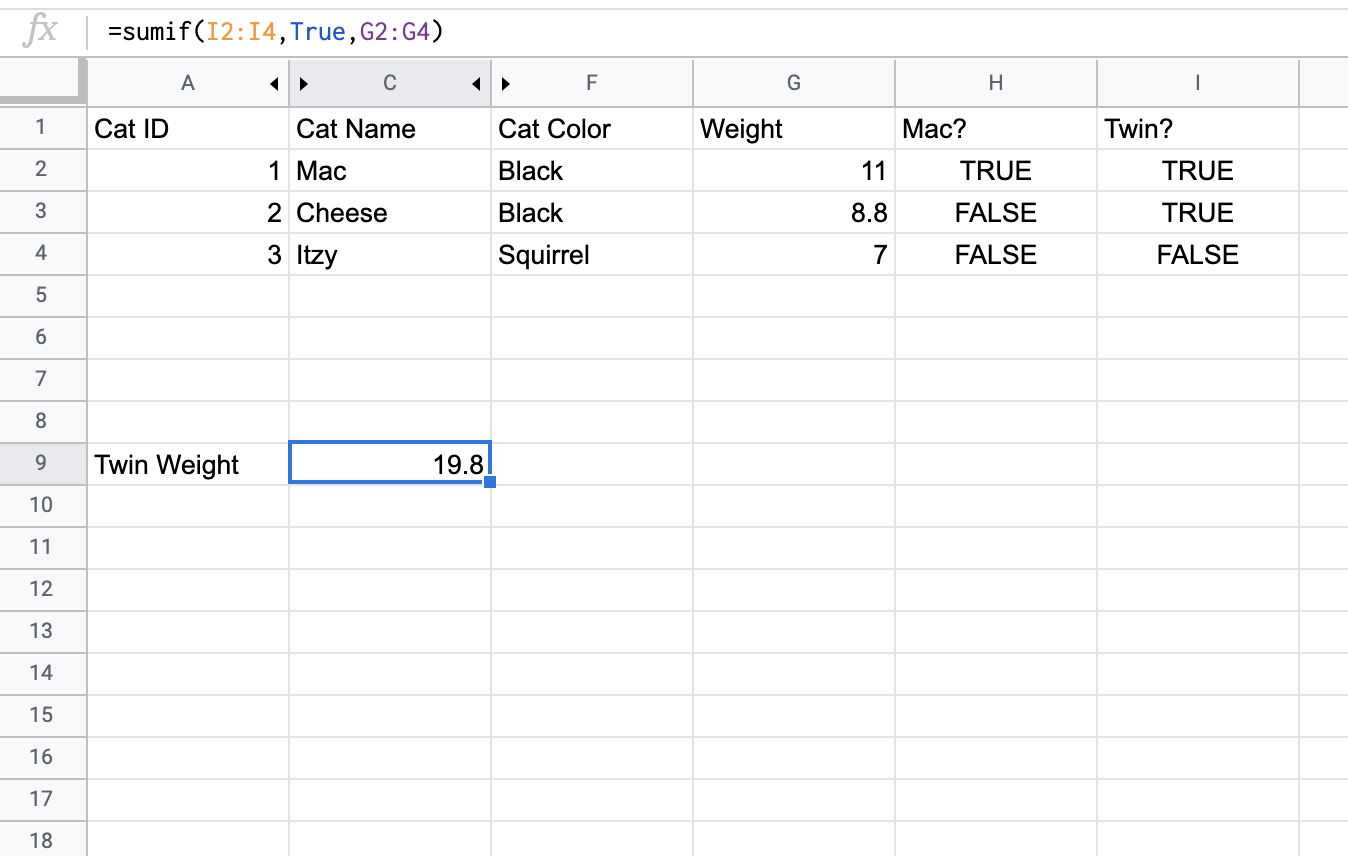The twins total weight is almost 20 pounds!

``````SELECT
CASE
WHEN cat_name = 'Mac' OR cat_name = 'Cheese'
THEN TRUE
ELSE FALSE
END AS is_twin,
SUM(weight) AS total_weight
FROM cat_table
GROUP BY is_twin;
``````

This requires a bit of explanation. First we are using a `case` statement to label whether a cat is a twin or not. Then we sum the weights of cats grouped by is_twin. This will give us:

IS_TWIN TOTAL_WEIGHT
TRUE 19.8
FALSE 7

The results are not formatted the same as a SUMIF since we also get the non-twins weights, but we get the data we need: 19.8 pounds of mini demon panthers sent here to ruin my couch.

This query can also be written as

``````SELECT
SUM(weight) AS total_weight
FROM cat_table
WHERE cat_name = 'Mac' OR cat_name = 'Cheese'
;
``````

This will simply give you 19.8.

### COUNTIF

If we want to count the number of female and male kittens we have, in Excel, we would do:
`=countif(M1:M4, "Female")` and
`=countif(M1:M4, "Male")`Similar to the total_weight calculation above, this can be accomplished in SQL by:

``````SELECT
gender,
COUNT(*) AS count
FROM cat_table
GROUP BY gender
``````

This will give us

GENDER COUNT
male 1
female 2

Note that Snowflake actually does have a count if: https://docs.snowflake.com/en/sql-reference/functions/count_if.html

``````SELECT
COUNTIF(gender = 'Female') AS female_count
FROM cat_table
``````

I never use that function but maybe I’ll remember to now.

## SUBSTITUTE

Let’s say we got user entered info for our cat named and they appeared like this: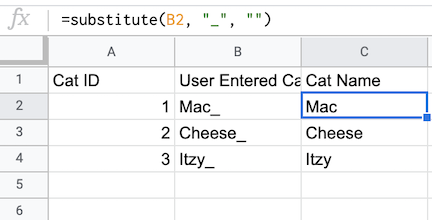We would want to clean it up by removing the _, essentially replacing it with a blank string. We would do a substitute like this:
`=substitute(B2, "_", "")`

In Snowflake, we do a replace function. With replace, if we want to eliminate, we can enter only two arguments if we want to replace the substring with the empty string:

``````SELECT
REPLACE(user_entered_cat_name, '_') AS cat_name
FROM cat_table
``````

Similar to in Excel, if you want to replace multiple strings, you have to embed the REPLACE function:

``````SELECT
REPLACE(REPLACE(user_entered_cat_name, '_'), '!') AS cat_name
FROM cat_table
``````

It can end up being a really nasty line of code.

## CONCATENATE

To conCATenate in Excel (ha, get it), you use &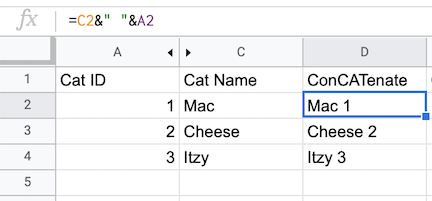In Snowflake, you use || or the CONCAT function

No matter which one you do, remember the spaces:

``````SELECT
cat_name || ' ' || cat_id,
CONCAT(cat_name, ' ', cat_id)
FROM cat_table
``````

## VLOOKUP / HLOOKUP

I know often in Excel, in order to combine data from multiple tabs, people do a VLOOKUP or an HLOOKUP. In databases, a VLOOKUP is basically a JOIN. An HLOOKUP is not really a thing. I’ll explain why.

### VLOOKUP

Let’s say someone gave us the cats cuteness levels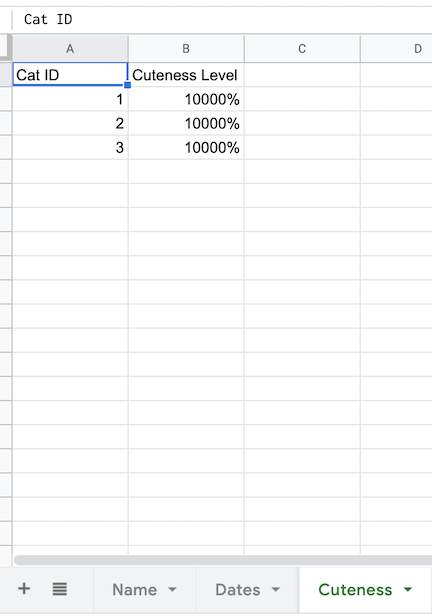To find the cats cuteness level, we do a VLOOKUP on Cat ID

`=VLOOKUP(A2,Cuteness!A:B,2,True)`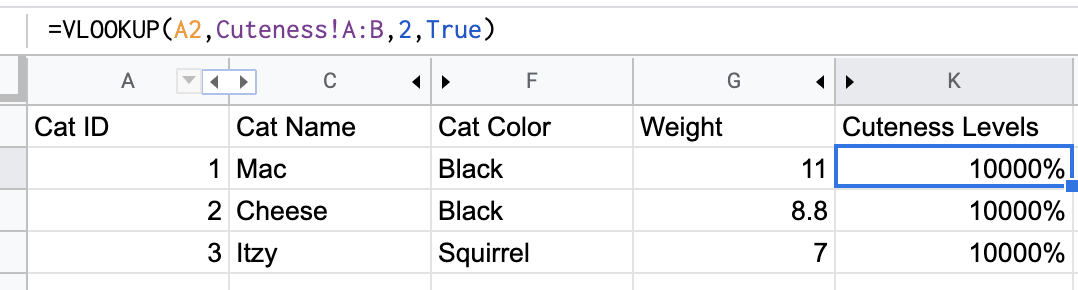Perfect, the cats are all top level cuteness. Notice that the vlookup is “on” a certain field. This is the field that the two datasets have in common; more specifically, the field that has to be equal in order for the data to be relevant for the row. To do this in databases, we join:

``````SELECT
*
FROM cat_info
LEFT JOIN cat_cuteness
ON cat_info.cat_id = cat_cuteness.cat_id
``````

Notice that we are joining “on” cat_id. In SQL, you can join on multiple fields (or none! don’t do that!). It’s most common to join on an ID field though.

Notice also that I used a LEFT join. Unlike in a VLOOKUP, we can choose what to include in the final data set. Had I used INNER JOIN, any cat_info without a cat_id in cat_cuteness would not have shown up in the data set. A RIGHT join would be the reverse of this; it would be like doing the VLOOKUP on the cat_cuteness tab.

### HLOOKUP

To be honest I haven’t used Excel in so long I couldn’t find a reason why you would need an HLOOKUP, except that someone who hates you gave you data. That’s okay. It happens.Let’s say someone gave you that. In order to get the info on to the main tab, we have to do:
`=hlookup(A2,'Cuteness, but someone who hates us gave it to us'!\$A\$1:\$D\$3,2,false)`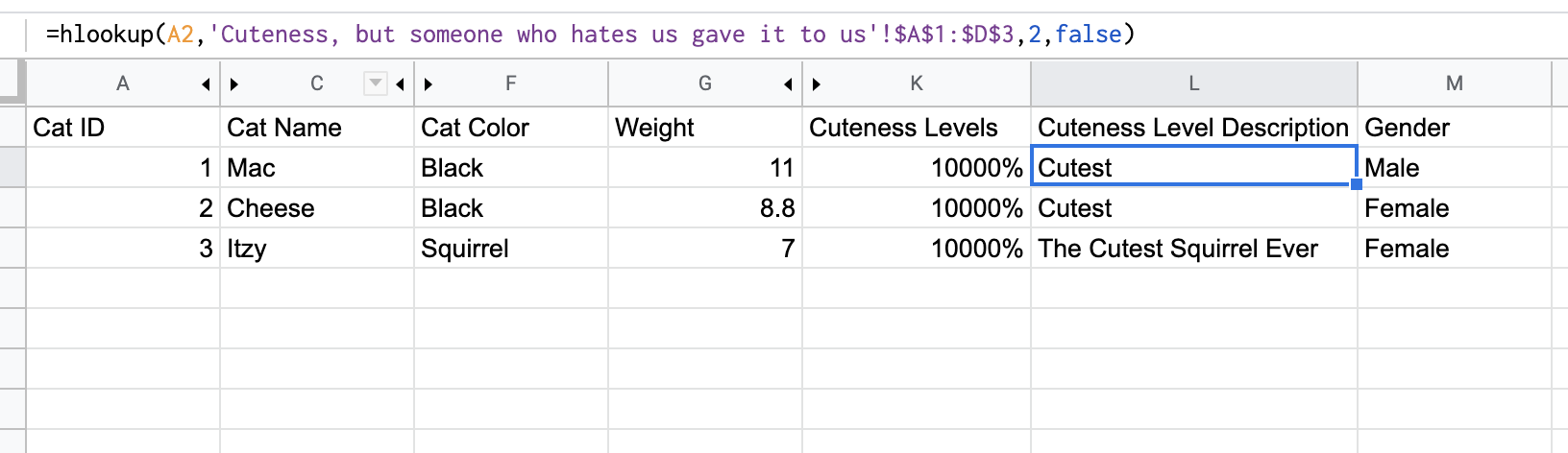There is no real hlookup in databases, because in databases, all tables and entities have to be formatted in the same way (with the column names being the first row).

If you can think of an example of HLOOKUP that you would be able to do in a database, let me know.